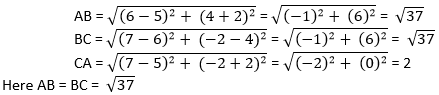Newbie

# Check whether (5, – 2), (6, 4) and (7, – 2) are the vertices of an isosceles triangle. Q.4

• 0

How i solve coordinate geometry question of class 10 easily  of exercise 7.1 ,  give the best solution of this question .Check whether (5, – 2), (6, 4) and (7, – 2) are the vertices of an isosceles triangle.

Share